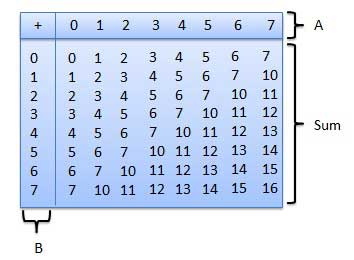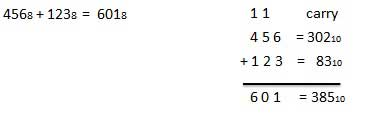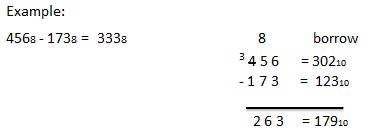# Octal Arithmetic

## Octal Number System

Following are the characteristics of an octal number system.

• Uses eight digits, 0,1,2,3,4,5,6,7.

• Also called base 8 number system.

• Each position in an octal number represents a 0 power of the base (8). Example: 80

• Last position in an octal number represents an x power of the base (8). Example: 8x where x represents the last position - 1.

### Example

Octal Number − 125708

Calculating Decimal Equivalent −

Step Octal Number Decimal Number
Step 1 125708 ((1 × 84) + (2 × 83) + (5 × 82) + (7 × 81) + (0 × 80))10
Step 2 125708 (4096 + 1024 + 320 + 56 + 0)10
Step 3 125708 549610

Note − 125708 is normally written as 12570.To use this table, simply follow the directions used in this example: Add 68 and 58. Locate 6 in the A column then locate the 5 in the B column. The point in 'sum' area where these two columns intersect is the 'sum' of two numbers.

```68 + 58 = 138.
```